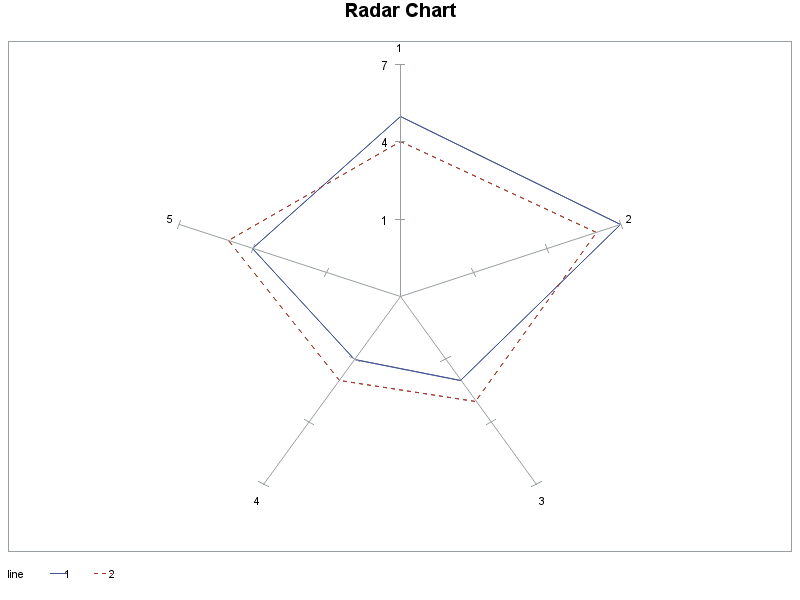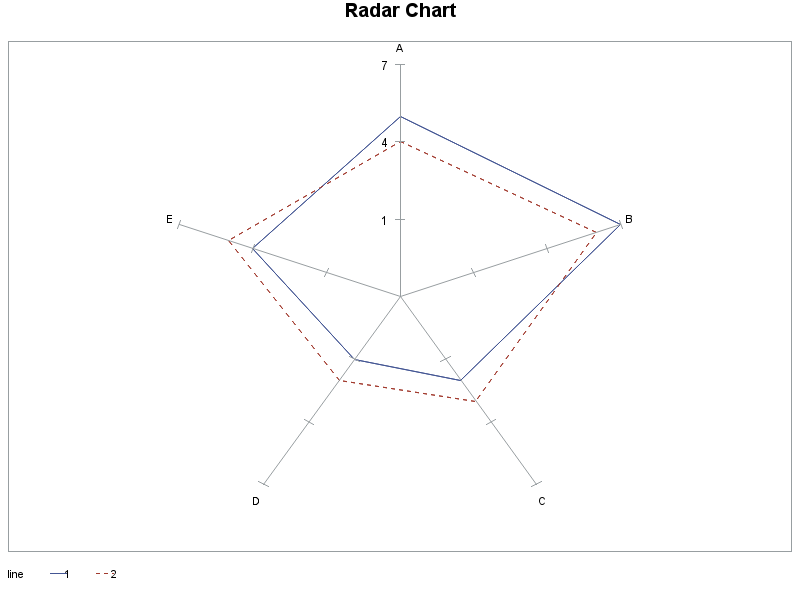## How do you modify the labels on a star plot using GCHART

Hello,

I am using the STAR command in GCHART to plot my data, which all works fine.

The default is to label the spines on the plot with the variable (call it X) used to create the spine.

I would like the chart to show a different label than X (call it Y).  Is that possible in GCHART?

For example, I would like the splines to correspond the the variable X which has the values "a" "b" "c" "d", but label the

spline with a very different variable Y which takes on different values.

Any help would be appreciated.

Dan

2 REPLIES 2

## Re: How do you modify the labels on a star plot using GCHART

If it's one to one mapping from X to Y, a format may be one way to do it easily.

## Re: How do you modify the labels on a star plot using GCHART

Here's some code to 'flesh out' what Reeza suggested.

Basically if you have a numeric variable X that you want to use to control the order of the radar chart spokes, but you have a character variable Y that you want to use to label the spokes (rather than using the X-values), you can create a user-defined format so that the x values will print as the y-text.

title;

data foo;
length y \$1;
input x y line value;
datalines;
1 A 1 5
2 B 1 7
3 C 1 2
4 D 1 1
5 E 1 4
1 A 2 4
2 B 2 6
3 C 2 3
4 D 2 2
5 E 2 5
;
run;

proc sql;
create table temp as
select unique x as start, y as label
from foo;
quit; run;
data control; set temp;
fmtname = 'xfmt';
type = 'N';
end = START;
run;
proc format lib=work cntlin=control;
run;

chart x / overlay=line freq=value;
run;

format x xfmt.;
chart x / overlay=line freq=value;
run;

The first graph is the radar chart with the spokes labeled with the numeric X, and in the 2nd chart the spokes are labeled with the Y text (via the user-defined format):Discussion stats
• 2 replies
• 711 views
• 1 like
• 3 in conversation